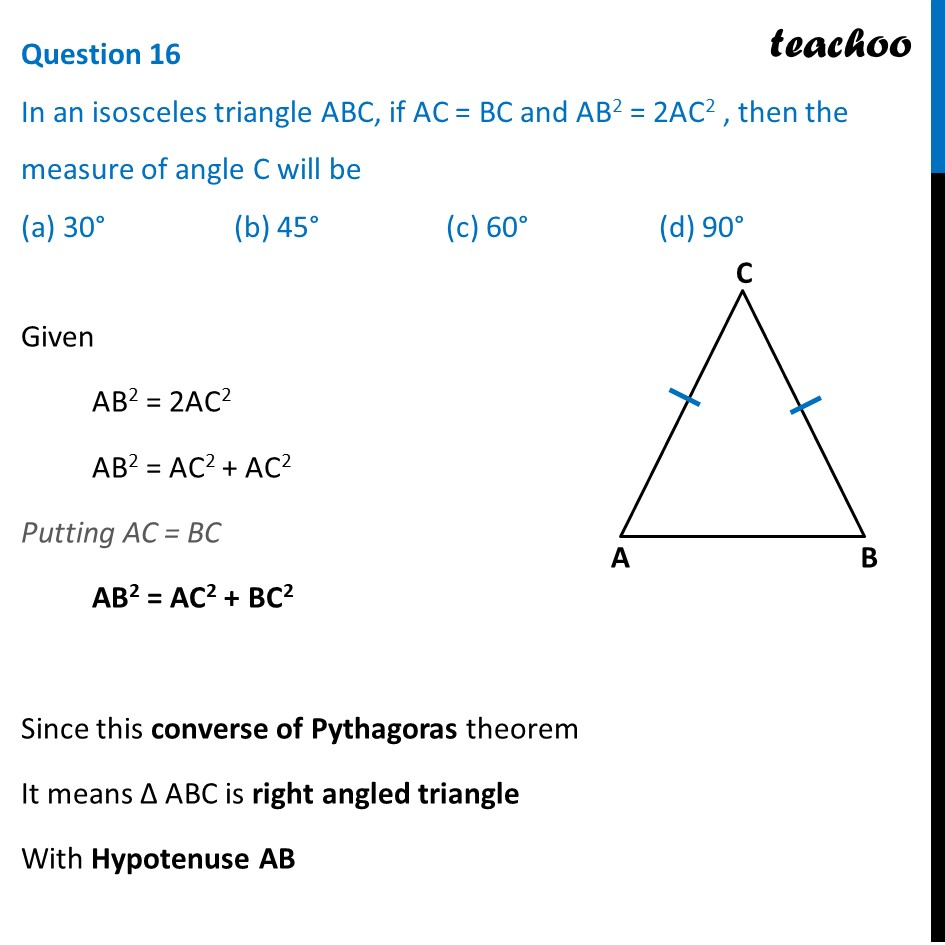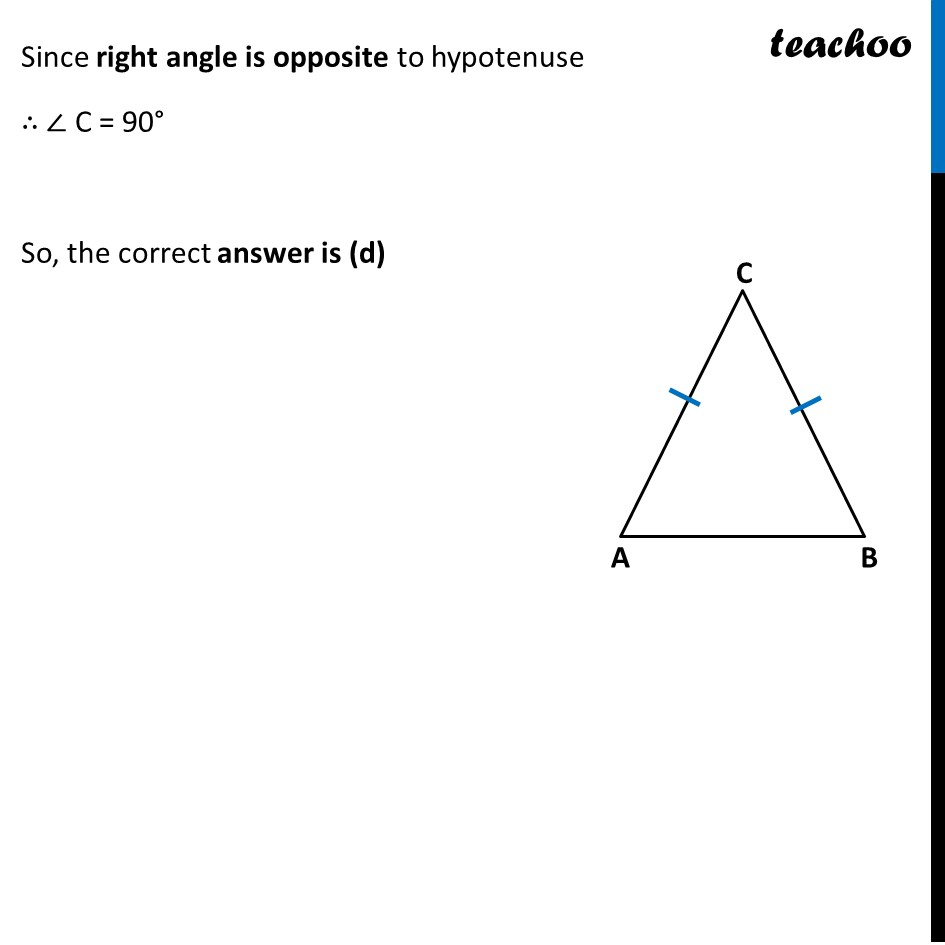## (a) 30°   (b) 45°   (c) 60°   (d) 90°

This question is inspired from Ex 6.5, 5 - Chapter 6 Class 10 - Triangles1. Class 10
2. Solutions of Sample Papers for Class 10 Boards
3. CBSE Class 10 Sample Paper for 2022 Boards - Maths Basic [MCQ]

Transcript

Question 16 In an isosceles triangle ABC, if AC = BC and AB2 = 2AC2 , then the measure of angle C will be (a) 30° (b) 45° (c) 60° (d) 90° Given AB2 = 2AC2 AB2 = AC2 + AC2 Putting AC = BC AB2 = AC2 + BC2 Since this converse of Pythagoras theorem It means Δ ABC is right angled triangle With Hypotenuse AB Since right angle is opposite to hypotenuse ∴ ∠ C = 90° So, the correct answer is (d)